# SilverZone - IOM PDF Sample Papers for Class 6

Class 6 sample paper & practice questions for International Olympiad of Mathematics (IOM) level 1 are given below. Syllabus for level 1 is also mentioned for these exams. You can refer these sample paper & quiz for preparing for the exam.#### Resources:

##### Sample Questions from Olympiad Success:
 Q.1 Q.2 Q.3 Q.4 Q.5 Q.6 Q.7 Q.8 Q.9 Q.10
 Q.1 Rahul distributed 2/3 of his marbles to his friends. If he had a total of 12 marbles, then how many marbles he distributed? a) 8 b) 6 c) 4 d) 3
 Q.2 If the area of rectangle increases from 2 sq cm to 4 sq cm then the perimeter will be: a) Decrease b) Increase c) Remains same d) None of these
 Q.3 There are 100 teachers in a school for 3000 students. Which of the following is the teacher student ratio? a) 3:100 b) 1:30 c) 1:1 d) 30:1
 Q.4 For 90 which symbol is used in Roman system? a) LX b) XC c) LXXX d) C
 Q.5 If 2x + 3 = 5, which of the following is the value of ‘x’? a) -4 b) 4 c) -1 d) 1
 Q.6 Which of the following is a prime number? a) 93 b) 81 c) 33 d) 97
 Q.7 In the given figure, the ray will be named as ______.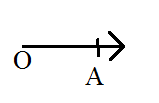a)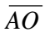b)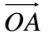c)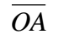d)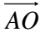Q.8 Form the equations: Sum of 2 times x and 3 times y is 1. a) 2x = 3y + 1 b) 3x + 2y = 1 c) 2x + 3y = 1 d) 3x = 2y + 1
 Q.9 Javed was given 5/7 of a basket of oranges. What fraction of oranges was left in the basket? a) 4/7 b) 5/7 c) 2/7 d) 12/7
 Q.10 Ram’s bank balance is Rs. 500 more than 3 times his friend’s bank balance. If his friend’s bank balance is ‘ y ‘. Then what is Ram’s bank balance? a) y + 500 b) 3y - 500 c) 3y d) 3y + 500Sample PDF of SilverZone - International Olympiad of Mathematics (IOM) PDF Sample Papers for Class 6:

 Q.1 )a Q.2 )b Q.3 )b Q.4 )b Q.5 )d Q.6 )d Q.7 )b Q.8 )c Q.9 )c Q.10 )d

Q.1 : a | Q.2 : b | Q.3 : b | Q.4 : b | Q.5 : d | Q.6 : d | Q.7 : b | Q.8 : c | Q.9 : c | Q.10 : d